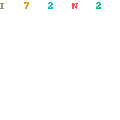# Mathematical Analysis and the Mathematics of ComputationMathematical Analysis and the Mathematics of Computation by Werner Römisch
English | PDF | 2016 | 722 Pages | ISBN : 3319427539 | 8.53 MB

This book is a comprehensive, unifying introduction to the field of mathematical analysis and the mathematics of computing. It develops the relevant theory at a modern level and it directly relates modern mathematical ideas to their diverse applications.

The authors develop the whole theory. Starting with a simple axiom system for the real numbers, they then lay the foundations, developing the theory, exemplifying where it’s applicable, in turn motivating further development of the theory. They progress from sets, structures, and numbers to metric spaces, continuous functions in metric spaces, linear normed spaces and linear mappings; and then differential calculus and its applications, the integral calculus, the gamma function, and linear integral operators. They then present important aspects of approximation theory, including numerical integration. The remaining parts of the book are devoted to ordinary differential equations, the discretization of operator equations, and numerical solutions of ordinary differential equations.

This textbook contains many exercises of varying degrees of difficulty, suitable for self-study, and at the end of each chapter the authors present more advanced problems that shed light on interesting features, suitable for classroom seminars or study groups. It will be valuable for undergraduate and graduate students in mathematics, computer science, and related fields such as engineering. This is a rich field that has experienced enormous development in recent decades, and the book will also act as a reference for graduate students and practitioners who require a deeper understanding of the methodologies, techniques, and foundations.